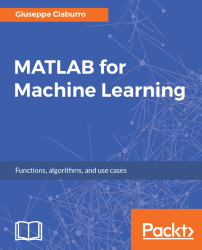•#### MATLAB for Machine Learning#### Overview of this book

MATLAB is the language of choice for many researchers and mathematics experts for machine learning. This book will help you build a foundation in machine learning using MATLAB for beginners. You’ll start by getting your system ready with t he MATLAB environment for machine learning and you’ll see how to easily interact with the Matlab workspace. We’ll then move on to data cleansing, mining and analyzing various data types in machine learning and you’ll see how to display data values on a plot. Next, you’ll get to know about the different types of regression techniques and how to apply them to your data using the MATLAB functions. You’ll understand the basic concepts of neural networks and perform data fitting, pattern recognition, and clustering analysis. Finally, you’ll explore feature selection and extraction techniques for dimensionality reduction for performance improvement. At the end of the book, you will learn to put it all together into real-world cases covering major machine learning algorithms and be comfortable in performing machine learning with MATLAB.
Title PageCreditsForewordwww.PacktPub.comCustomer FeedbackPrefaceFree Chapter
Getting Started with MATLAB Machine LearningImporting and Organizing Data in MATLABFrom Data to Knowledge DiscoveryFinding Relationships between Variables - Regression TechniquesPattern Recognition through Classification AlgorithmsIdentifying Groups of Data Using Clustering MethodsSimulation of Human Thinking - Artificial Neural NetworksImproving the Performance of the Machine Learning Model - Dimensionality ReductionMachine Learning in Practice## Describing differences by discriminant analysis

Discriminant analysis is a statistical analysis dating back to Fisher (1936 - Linear Discriminant Analysis (LDA)), as we have already mentioned earlier. It is a method for describing, through a one-dimensional function, the difference between two or more groups and allocating each observation to the group of origin. This is a classification problem, where the groups are known a priori and one or more new observations are classified into one of the known groups based on the measured characteristics. Each observation must have a score on one or more quantitative predictor measures, and a score on a group measure. Discriminant analysis is useful in determining whether a set of variables is effective in predicting category membership.

In MATLAB, the discriminant analysis model is created on the following assumptions:

• Each class generates data using a multivariate normal distribution. That is, the model assumes that data generated has a Gaussian mixture...